### A simple example of a frequency filter usage

We will illustrate the use of frequency filters in the simple and often applied case where the noise is much more broadband than the signal.  We consider a somewhat composite signal which has the following mathematical shape:

s(t) = cos(3.02*t).*sin(20.81*sin(t)+2*t)

The function can be thought of in mV, and the time in seconds, Milli seconds or micro seconds.  In most of the illustrations below, we will take time to be given in seconds, which makes for a very low frequency signal.  We can think of it as the signal from, say, a mechanical sensor that describes some or other motion.  We took this somewhat involved expression to have a certain wealth in spectral composition.

A small piece of it looks like this: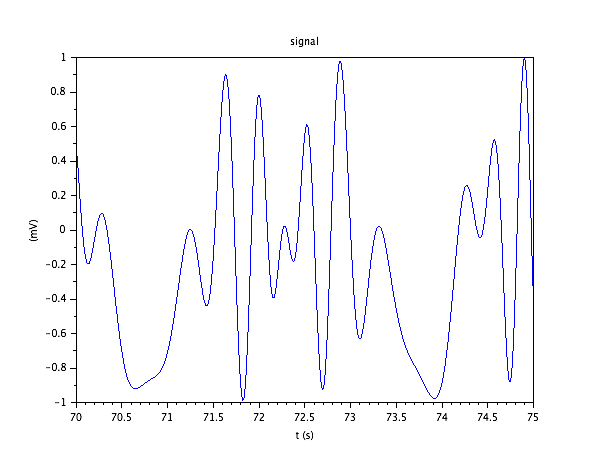The spectral density (in arbitrary units - because in reality these are Dirac peaks) looks like: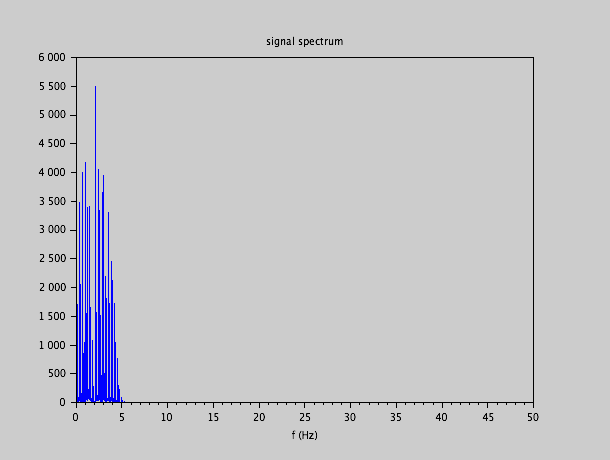We see that the spectral content of the signal is between 0 Hz and 5 Hz somewhere.  Also, that the highest frequencies in this range have relatively low amplitudes.  This is in fact the only information that we will use about our signal, in order to design a frequency filter: we will use the fact that the spectral energy of the signal is in the interval 0 Hz - 5 Hz.

We now consider that we add noise to the signal, and that it is only this noisy variant that is available to us.  The noise is "white" with an RMS value of 1 mV.  In reality the noise will have a bandwidth of up to 50 Hz in this example.  So the signal we will have to treat has the following look: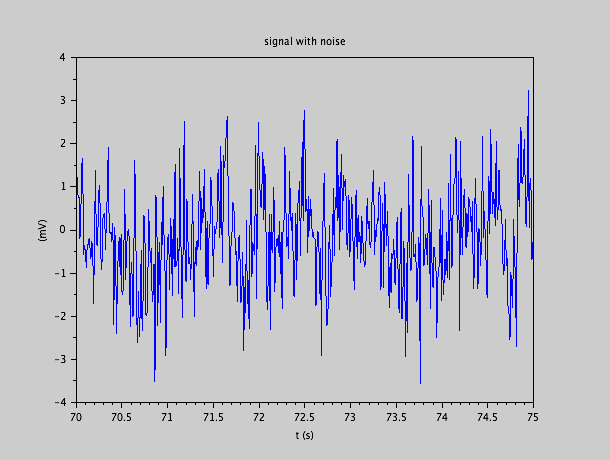Obviously, by eye, we hardly recognize the original signal we would like to extract.

When looking at the spectral content of this noisy signal, we get the following spectrum: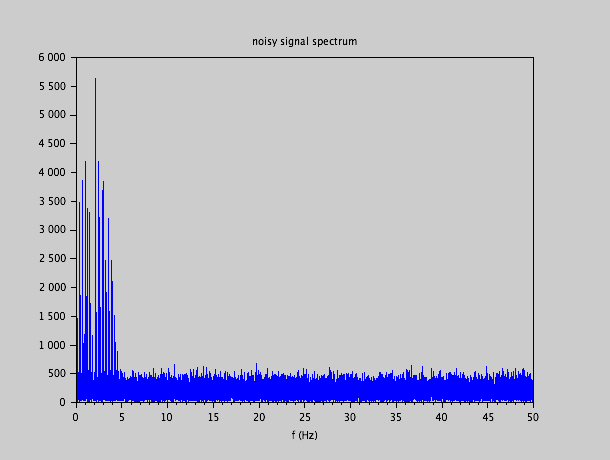We see here clearly that the spectrum of the original signal stands out over the white noise spectrum.  Nevertheless, there will be a significant contamination due to noise within the band of the signal itself: we will not be able to restore the original signal completely if our only criterion to distinguish noise from signal is the selection of the useful band of the signal: the noise in that band will remain in the output signal of course.  We also notice that although the original signal had a bandwidth of 5 Hz, there is more noise than signal between 4 and 5 Hz.  As such, the signal will only come out of the noise in the band from 0 to 4 Hz, so this is the part of the spectrum that we want to keep.  If we use a perfect filter which keeps everything below 4 Hz and rejects everything at higher frequencies, we obtain the following restored signal from the noise as compared with the original signal: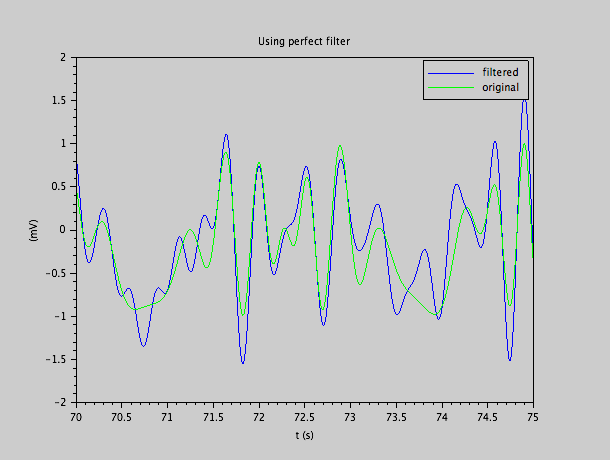Although the restored (blue) signal is not identical to the original (green) signal, which was expected as there was noise in this band too, we nevertheless have a much closer signal to the original than the noisy signal showed earlier, where one couldn't even recognize the rough shape of the original signal.  Let us say that using a perfect filter, this is the best we can do to extract the original signal out of that noisy signal if we want to limit ourselves to frequency filters - that is, if we want to use as only information about the original signal, the band where it is useful.

Note that we limited somewhat the signal bandwidth: we went down from the 5 Hz the full signal covers, to 4 Hz for the filter.   The signal spectrum between 4 and 5 Hz is hence also lost.  We did this, because in this band, there was more noise than signal, so there was nothing to recover.  But did we distort the signal much by cutting away part of its spectrum ?  We can verify this by filtering the original signal without noise with the 4 Hz filter, and see how things differ: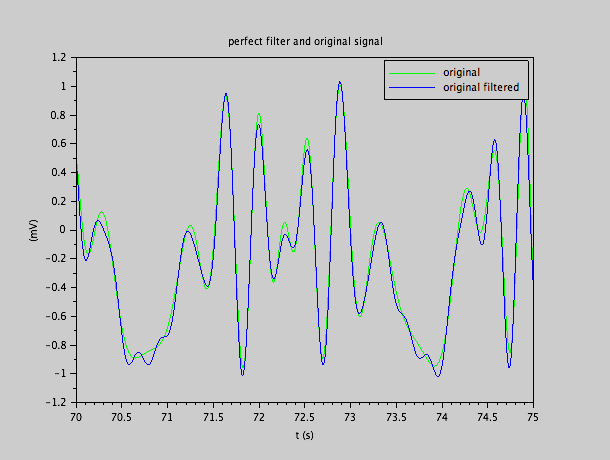Note that the filtered signal has some small wiggles extra (between 70.5 and 71 seconds for instance, or between 73.5 and 74 seconds).  Nevertheless, we see that filtering at 4 Hz doesn't significantly alter the signal.  As such, the deviations we saw earlier were the result of noise, and not of the fact that the filter was cutting some signal away.

One might wonder why one would bother with any filter approximations, if the perfect filter is possible (as we did right here).  The point is that the perfect filter is possible once we have the entire signal.   Then we take the Fourier transform, put the part above 4 Hz to 0, and do the inverse Fourier transform.  Obviously we then have the part of the signal that was below 4 Hz, unaltered, and we cut away the components above 4 Hz entirely.

A real filter has to treat the signal as it is coming in, not when we have received it entirely.  We implemented simulations of 3 classical filters: a Butterworth filter, cutting off at 4 Hz ; a Chebyshev I filter, cutting off at 4 Hz, and allowing for a ripple of 10% of the amplitude in the pass band ; an elliptic (Cauer) filter with a cutoff at 4 Hz, a ripple in the pass band of 10%, and a suppression in the stop band of at least 1%.  We limited ourselves to filters of order 3.

The result of these filters on the noisy signal can be seen here: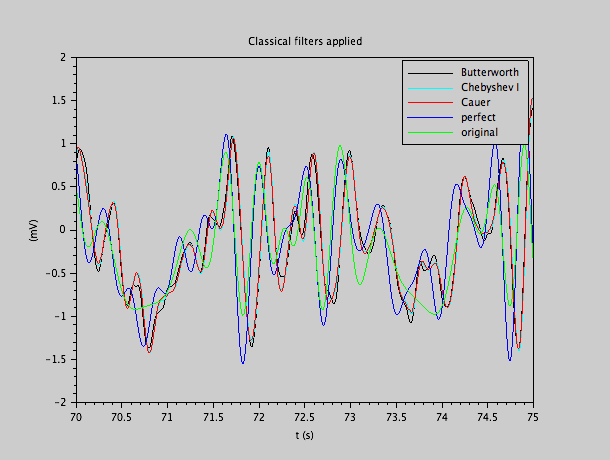The first thing that we note, when we compare the outputs of these filters to the original signal or to the signal that went through the perfect filter, is a delay.  Indeed, causal filters will give their output with some delay, here, of the order of about 0.2 seconds.    Next, we note that the three filters have very similar responses.  Only the Butterworth filter has some small deviation at some peaks from the two others ; this deviation is however small as compared to the deviation from the perfect filter, or from the original signal, so one cannot say that the Butterworth filter is less good than the other two filters.

The amplitude filtering of our three realistic filters looks like this: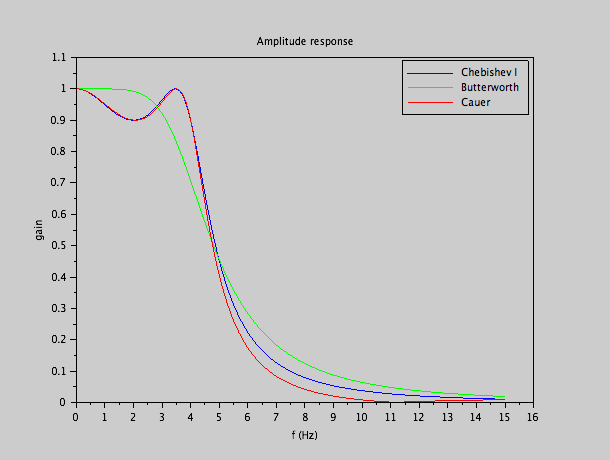The classical properties are recognized: the Butterworth filter has the slowest transition, but is the most horizontal at low frequencies.  The Chebyshev I type filter has an allowed ripple of 10% in the pass band, but cuts off much steeper.   The Cauer filter is even more steep ; however, the Cauer filter will increase in the stop band again, while the Chebyshev I filter will steadily decrease.

The illustrated case is a very simple, but frequent, usage of a frequency filter: we have a useful signal that is limited to a smaller band than a broad-band noise, and if the only information we have, or that we want to use, is this, then it is appropriate to filter the signal with a frequency filter that limits the pass band to the useful signal band.  As we see in this example, the filter doesn't have to be very sophisticated.   We see almost no difference in quality between the perfect filter, and third order classical filters.  There is no reason to build any sophisticated filter in this particular case, and the result is nevertheless impressive: from the noisy signal where nothing could be recognized, we find a reconstruction which, if not perfect, nevertheless has most of the features of the original signal recovered.

### Implementation

We can wonder whether we implement this filter in an analogue or a digital way.  The first reflex would be to do this with a digital filter.  Indeed, the frequencies involved are (in the original example), very low (less than 50 Hz).  Moreover, the dynamics of the useful signal over the noise is small.  We would hence need only a slow, low dynamics ADC to do this.  Simple controllers have this kind of ADC often on-board.  However, in order to go digital, one would in any case need a low-pass anti-aliasing filter.  One can wonder what would be the gain in building an anti-aliasing filter at, say, 20 KHz if the ADC runs at 40 Ks/s, in order to implement a digital filter at 4 Hz.  The question is in fact not so simple.  It might be that anti-aliasing filter at 20 KHz is easier to build than the filter at 4 Hz.  But of course if the filter at 4 Hz is of similar complexity and quality as the one at 20 KHz, then there's no point in doing this digitally.  The principal reason is, as we saw, that we don't need any sophisticated filter for the problem at hand.

If we do an analogue implementation, we have to decide between a passive and an active filter.

The synthesis for a passive filter can result in the following circuits:

The Cauer filter takes on the following shape: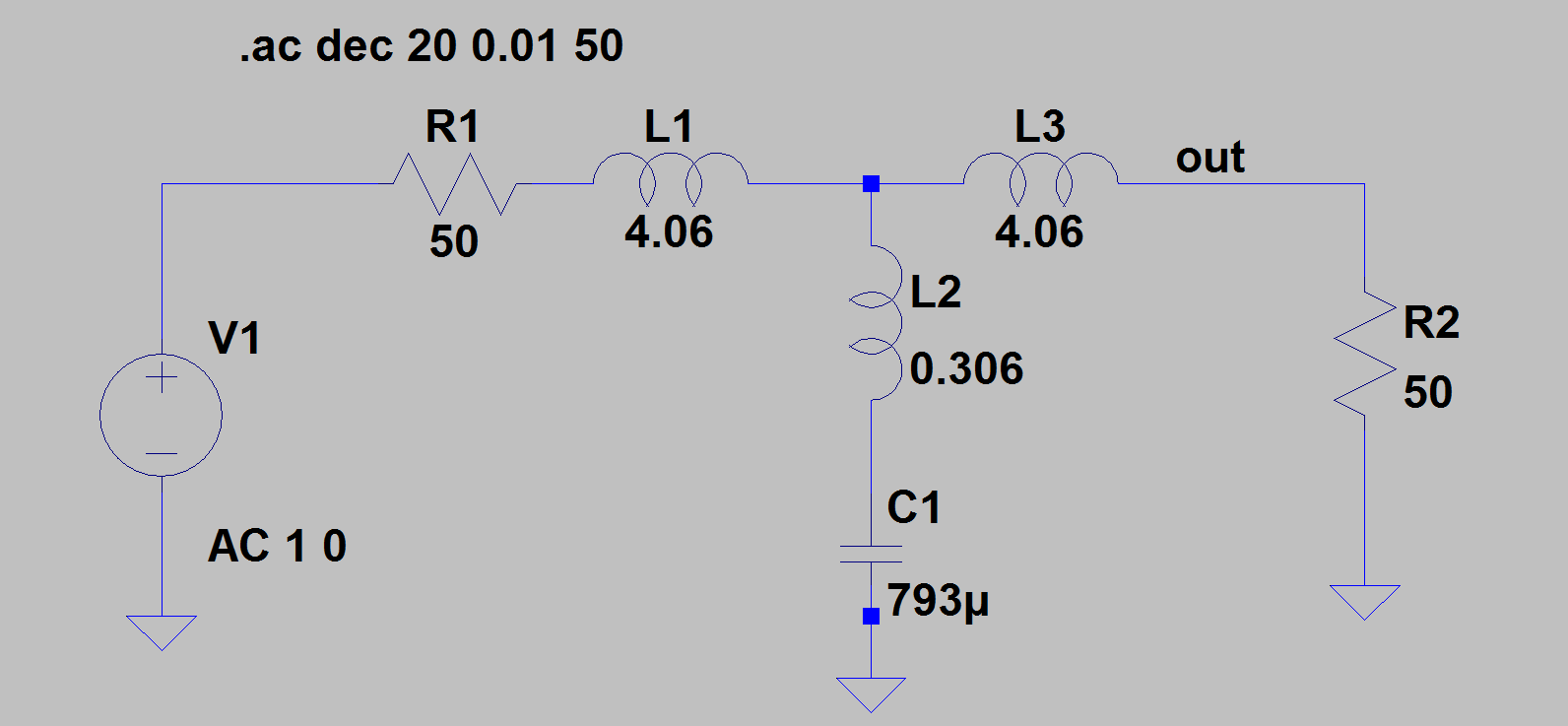while the Butterworth filter looks like this: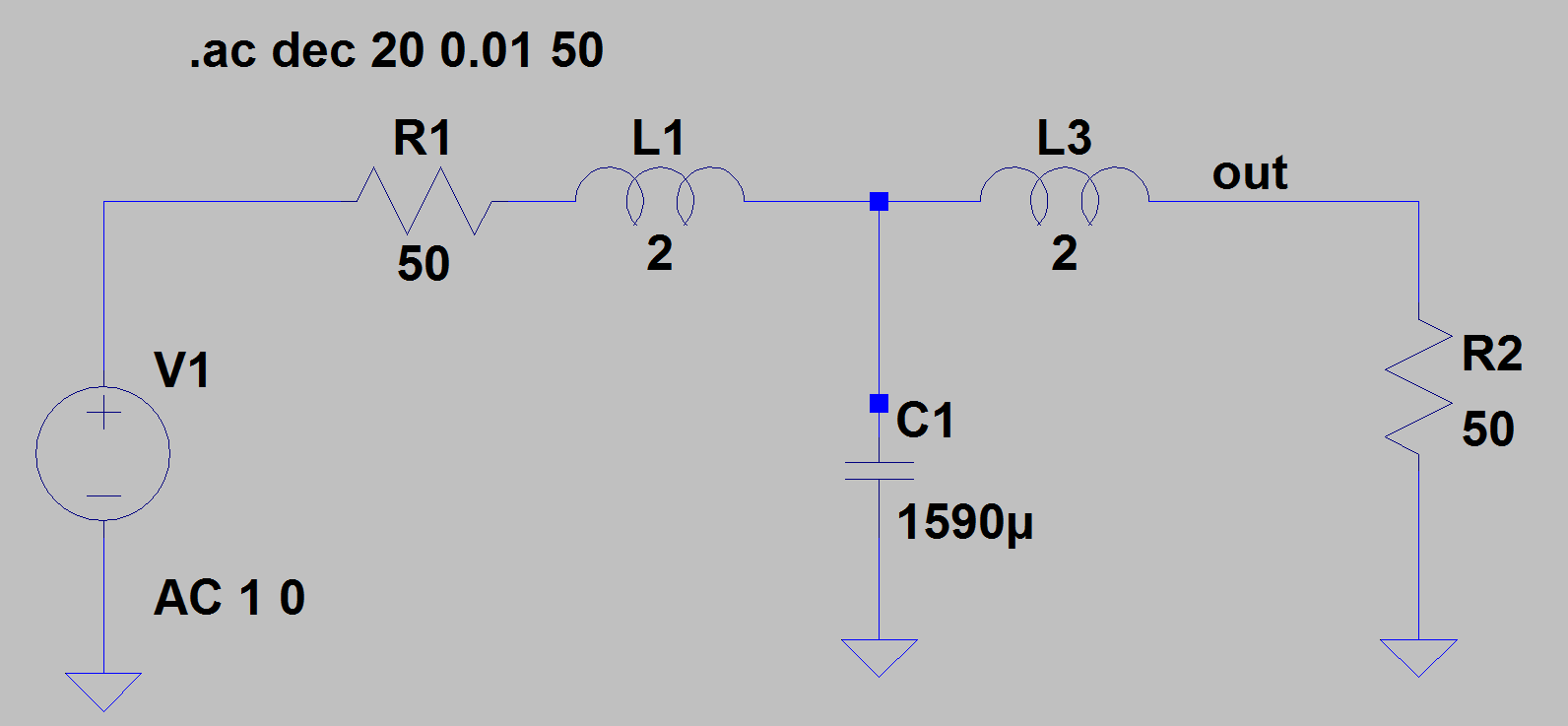As we can see, the components are not practical at all: the large values of the inductors and the capacitors make for expensive, bulky, and non-ideal circuits.  This is always the case for passive filters at very low frequencies.  Note that if the time unit were micro seconds, and the frequency axis in this example were given in MHz, then this filter would be very easily and practically be realisable, with capacitors in the nF range, and inductors in the micro Henry range.

If we go to active filter implementations, we can have an implementation of the Chebyshev I filter as follows: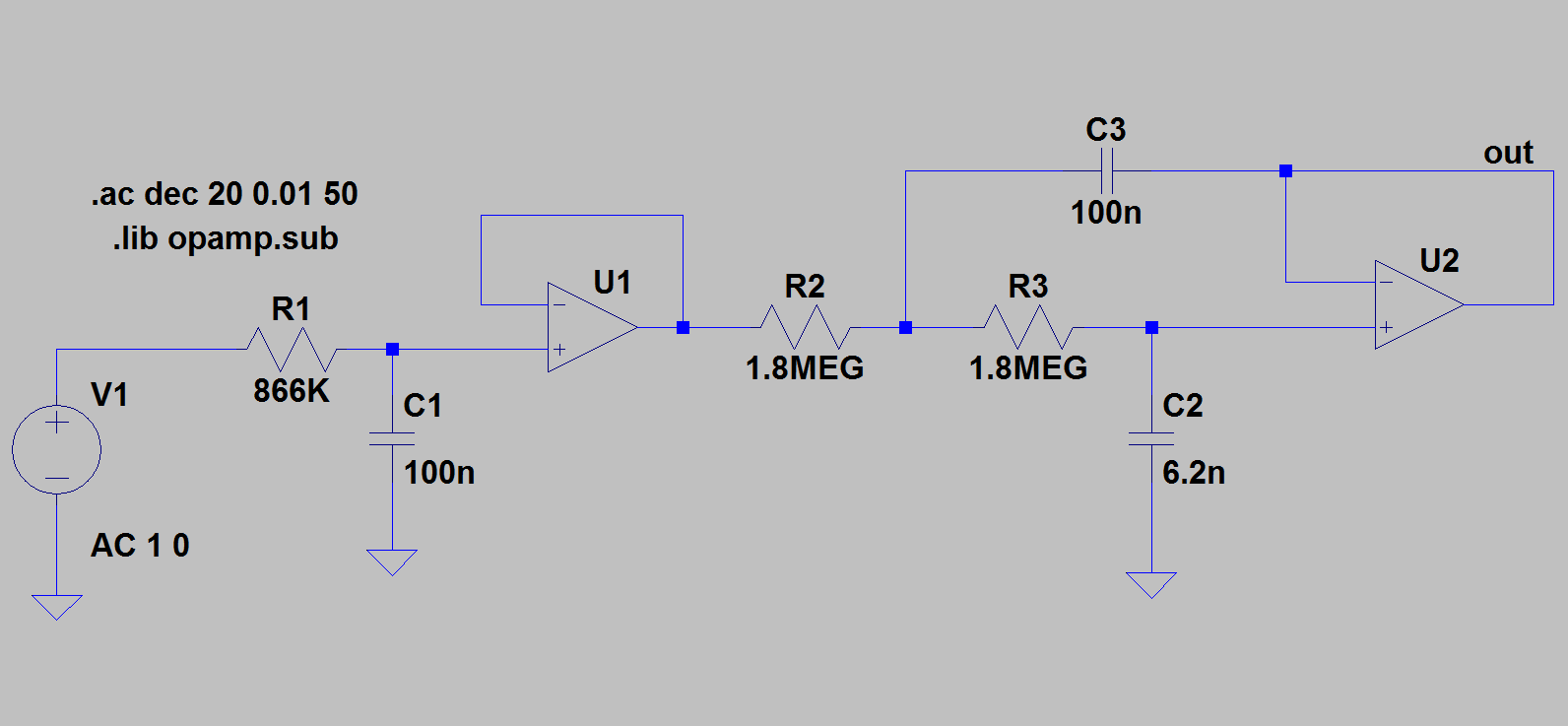As we see, the active filter is much easier to realize with reasonable components.

Even the Cauer filter can be implemented with not many more components: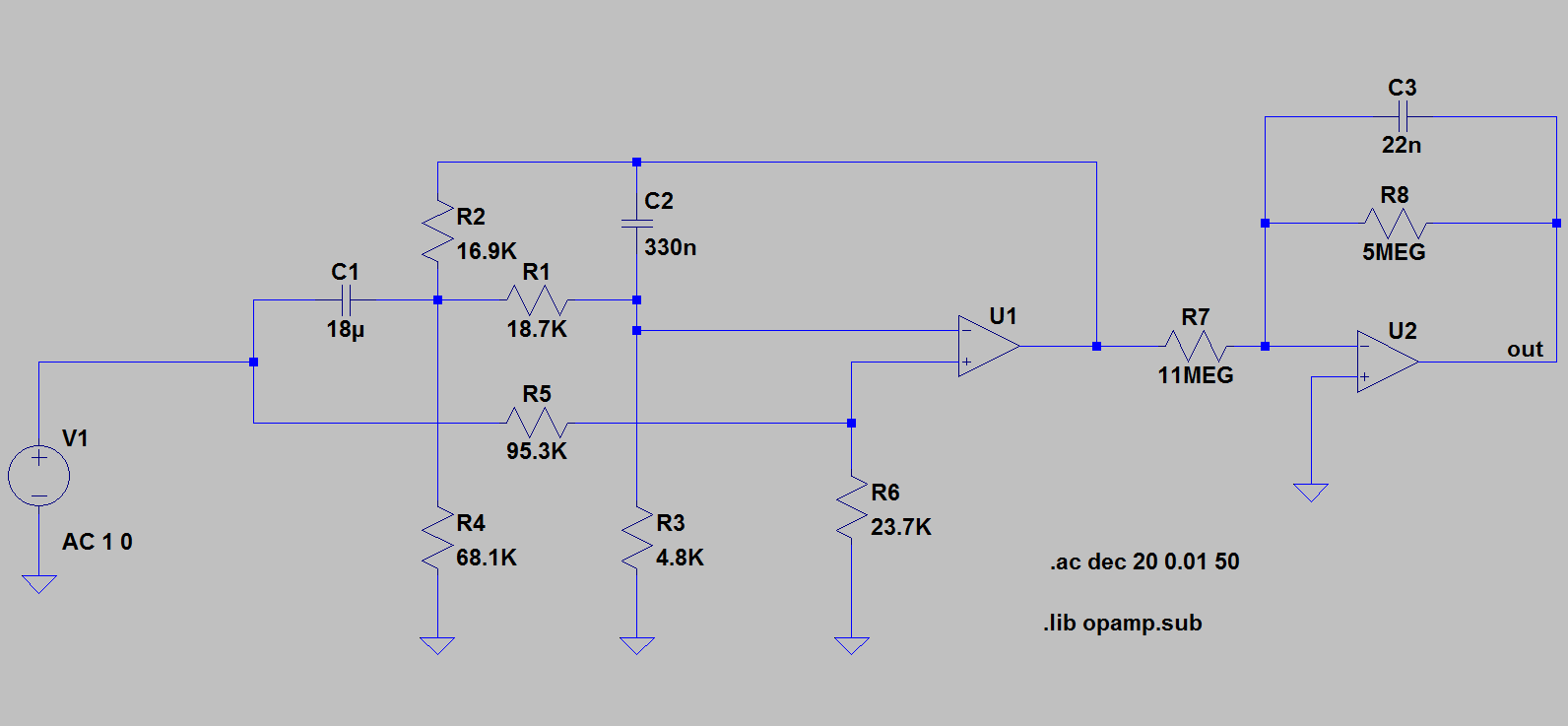We might also consider the digital filter option.  As we said before, we need in any case an analogue anti-aliasing filter, so it might seem useless, at first sight, to implement a digital filter to avoid an analogue filter, if we need in any case an analogue filter.  However, in this particular case, given the low cutoff frequency needed, even with a very weak anti-aliasing filter, we can find a solution.  Indeed, given that the cutoff frequency is 4 Hz, a first-order anti-aliasing filter (an RC filter) will cut off at 20 dB per decade.  At 4 KHz, it will hence cut off 60 dB, and at 400 Hz, it will cut off 40 dB exactly what we wanted of our Cauer filter in the stop band.   At a sample rate above 8 Ks/s, say, 10 Ks/s, we can hence use this simple RC filter as anti-aliasing filter, if we implement a digital filter that will cut off more severely at 4 Hz.

A sample rate of 10 Ks/s and the processing that goes with it can easily be handled by a simple controller.  However, the ratio between the sample frequency (10 KHz) and the cutoff frequency (4 Hz) is 2500.   That is the order of magnitude of the number of taps that a FIR filter would need (the "size" in time of the impulse response of the filter in units of sample time).  Let us say that a good filter would need 10 000 taps.   That would mean 10 000 multiplications and 10 000 additions per sample, or 200 million floating point operations per second.   That might stress a simple controller somewhat !  Of course such rates are possible with FPGA or DSP, but then we are using a more expensive system.

In reality, when the ratio between the sample frequency and the bandwidth of the filter is high, one needs to opt for an IIR filter (which will then have a response very close to its analogue part).  So we will not even consider any FIR design in this example.

A 4th order Butterworth filter would become this, where this code (in an undefined language) has to  be executed for each incoming sample:

`a1 = 0.9998;a2 = 3.9992;a3 = 5.9988;a4 = 3.9992;a5 = 0.9998;b1 = 0.9934540;b2 = - 0.9803406;b3 = 5.98039191;b4 = - 3.9934325;scale = 2.486e-12;in(5) = in(4);in(4) = in(3);in(3) = in(2);in(2) = in(1);in(1) = newin;outnew = a1 * in(5) + a2 * in(4) + a3 * in(3) + a4 * in(2) + a5 * in(1);outnew = outnew  - b1 * out(4) - b2 * out(3) - b3 * out(2) - b4 * out(1);outnew = scale * outnew;out(4) = out(3);out(3) = out(2);out(2) = out(1);out(1) = outnew;`

We can just as well implement an Elliptic filter with the above code, the only thing to change are the values of the a and b coefficients.

`a1 = 0.0099881;a2 = -0.0399512;a3 = 0.0599264;a4 = a2;a5 = a1;b1 = 0.9975666;b2 = -3.99269;b3 = 5.9926803;b4 = -3.9975568;scale = 1.0;`

As we see, contrary to the analogue implementation, the digital implementation of an elliptic our Cauer filter is not more complex than the implementation of a pure pole filter.  The reason is that Chebyshev and Butterworth filters are not pure pole in the digital domain in any case, and have finite zeros just like elliptic filters.

There where one would potentially build an analogue (passive or active) Butterworth or Chebyshev I filter for reasons of simplicity and economy, even if the transfer function of the elliptic filter would be better, there's no such trade-off to be made in the case of a digital filter.  Hence the higher interest of elliptic filters in the digital domain.

Note that both filters need about 20 floating point operations per sample (about 10 additions, and 10 multiplications).   This comes down to 200 000 floating point operations per second, which is well within reach of even the simplest controllers.  It can also be implemented without the slightest problem in the smallest FPGA, available for a few 10 of Euros, but then one still needs to set up the ADC separately.

### Conclusion

We illustrated a very frequent case of frequency filter usage: Limiting the bandwidth to the smaller useful portion of the spectrum when a relatively small bandwidth signal is contaminated with wide band noise.  We took an artificial very low frequency example, in which case an active filter or a digital filter was the better choice.  We also saw that we didn't need any high performance filter, as the noise bandwidth was much larger than the signal bandwidth, so most of the noise was eliminated, even with a simple filter.  We saw that an elliptic filter is more involved than a Butterworth or Chebyshev I filter in the analogue case, but that this doesn't matter for a digital filter.

This example didn't allow us to illustrate the usefulness of more powerful filters, or of passive filters.